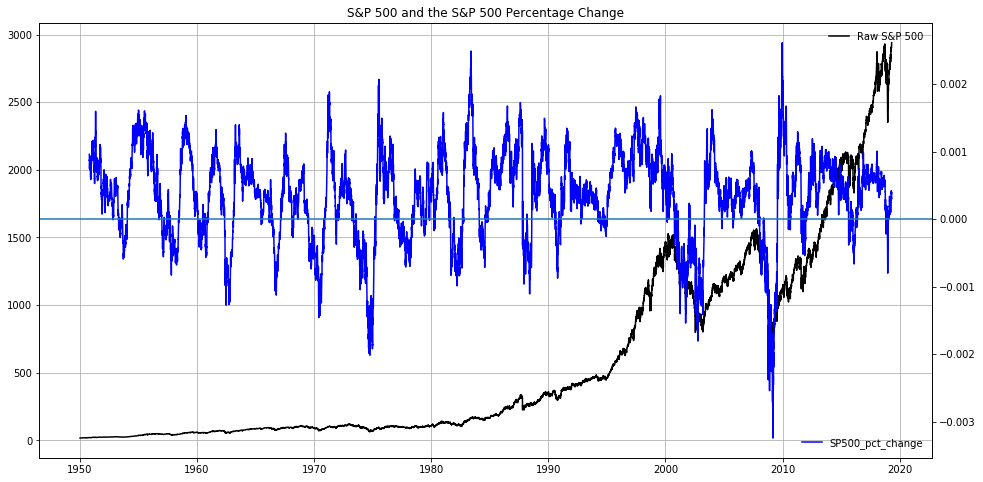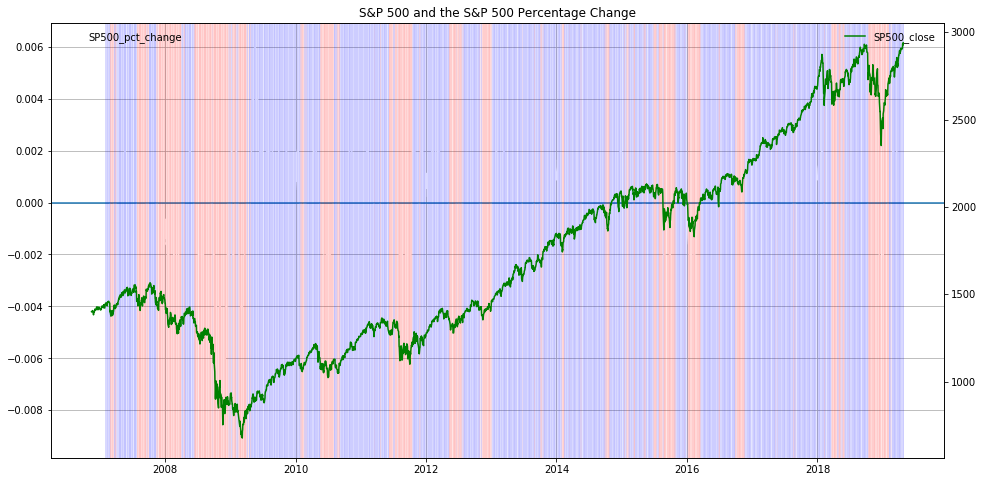### Get the "Applied Data Science Edge"!Fundamental Market Analysis with Python - Find Your Own Answers On What Is Going on in the Financial Markets

### Hot off the Press!My New Book: "The Little Book of Fundamental Analysis: Hands-On Market Analysis with Python" is Out!

### Grow Your Web Brand, Visibility & Traffic Organically5 Years of amunategui.github.Io and the Lessons I Learned from Growing My Online Community from the Ground Up

### Introduction

Lets color the background of a chart to enhance some aspect of a market. By using the axvline()” function of Matplotlibs Axis function, we can customize the color of every vertical line available. Obviously, as this is at the pixel level, we have to keep things simple to keep them legible.

### Code

Enhancing Moves with Background Coloring of the S&P 500
In :
from IPython.display import Image
Image(filename='double logos.png')

Out:In :
%matplotlib inline
import matplotlib
import matplotlib.pyplot as plt
import io, base64, os, json, re
import pandas as pd
import numpy as np
import datetime
import warnings
warnings.filterwarnings('ignore')


# Load S&P 500 Historical Data¶

In :
gspc_df = pd.read_csv('^GSPC.csv')
gspc_df['Date'] = pd.to_datetime(gspc_df['Date'])

gspc_df.columns = ['Date', 'SP500_close']
gspc_df['SP500_pct_change'] = gspc_df['SP500_close'].pct_change()

Out:
Date SP500_close SP500_pct_change
0 1950-01-03 16.66 NaN
1 1950-01-04 16.85 0.011405
2 1950-01-05 16.93 0.004748
3 1950-01-06 16.98 0.002953
4 1950-01-09 17.08 0.005889
In :
gspc_df.tail()

Out:
Date SP500_close SP500_pct_change
17436 2019-04-22 2907.969971 0.001012
17437 2019-04-23 2933.679932 0.008841
17438 2019-04-24 2927.250000 -0.002192
17439 2019-04-25 2926.169922 -0.000369
17440 2019-04-26 2939.879883 0.004685

# Simple Visualization using the Percentage Change¶

A great way of enhancing price action visuals and your understanding of what is going on is to color the background of the chart. By using the "axvline" of the Matplotlibe's Axis function, we can color every vertical line a custom color. Obviously, as this is at the pixel level, we have to keep things simple so that the colors are still legible.

In :
fig, ax = plt.subplots(figsize=(16, 8))
plt.plot(gspc_df['Date'], gspc_df['SP500_close'], color='black', label='Raw S&P 500')
plt.grid()
ax.legend(loc='upper right', frameon=False)
plt.title('S&P 500 and the S&P 500 Percentage Change')

# Get second axis
ax2 = ax.twinx()
plt.plot(gspc_df['Date'],
gspc_df['SP500_pct_change'].rolling(window=200).mean().values, color='blue', label='SP500_pct_change')
plt.axhline(0)
ax2.legend(loc='lower right', frameon=False)

Out:
<matplotlib.legend.Legend at 0x12308aeb8>In :
tmp = gspc_df.copy()
cut_off_date = '2006-11-16'
tmp = tmp[tmp['Date'] >= cut_off_date]

# smoot the percentage change with a rolling average
tmp['SP500_pct_change'] = tmp['SP500_pct_change'].rolling(window=50).mean().values
tmp['i'] = range(1,len(tmp)+1)

fig, ax = plt.subplots(figsize=(16, 8))
plt.plot(tmp['Date'], tmp['SP500_pct_change'], color='white', label='SP500_pct_change')
ax.legend(loc='upper left', frameon=False)
plt.grid()
plt.axhline(0)

# track whenever the percentage change is above or b
pos_1 = tmp[tmp['SP500_pct_change'] >= 0]['i']
neg_1 = tmp[tmp['SP500_pct_change'] < 0]['i']

for x in pos_1:
ax.axvline(tmp[tmp['i']==x]['Date'].values, color='blue',linewidth=1,alpha=0.05)
for x in neg_1:
ax.axvline(tmp[tmp['i']==x]['Date'].values, color='red',linewidth=1,alpha=0.05)

plt.title('S&P 500 and the S&P 500 Percentage Change')

# Get second axis
ax2 = ax.twinx()
plt.plot(tmp['Date'],
tmp['SP500_close'], color='green', label='SP500_close')

ax2.legend(loc='upper right', frameon=False)

Out:
<matplotlib.legend.Legend at 0x13000c390>### Show Notes

(pardon typos and formatting -
these are the notes I use to make the videos)